×

Search anything:

# Cache in Python

#### Software Engineering PythonWe have explored caching by demonstrating it with a Python code example where intermediate results are cached and improved the overall performance. This is done through functools.lru_cache in Python.

Before learning about Cache in Python, Let's first learn about What is Caching?

## What is Caching?

Caching is a part of the memoization technique to store results of already computed results rather than computing them again and again with a constraint on memory to use.

Let's take an example:

Here is an expression:

F(x,y) = 2x + 3y - 4

You have to calculate results for the given Co-ordinates (x,y) in an order:

(2,3), (4,5), (4,5), (2,3), (7,8), (4,5), (2,3), (4,5)

You will observe that pairs (2,3) and (4,5) appear repeatedly.

To solve this problem, You can use two different approaches:

### First Approach:

You can calculate one by one for each pair(x,y). But this approach leads to extra computation for already computed values.

### Second Approach:

You can store the results of each computation or if it's already computed, fetching the results directly from the memory. Well, this approach reduces the computation but leads to the usage of memory.

How can you solve this problem if billions of queries are there but didn't have that much memory to store the results?

Here, you need a Cache.

In this blog, you will read about LRU Cache with constrained maxSize which is an inbuilt tool of python.

## LRU Cache

Caches are basically a hash table. Every data that goes inside it is hashed and stored making it accessible at O(1).

LRU Cache is a cache object that deletes the least-recently-used items if the memory available to the cache is exceeded.

Let's again take an example:

Here is a Cache with a size to store three pairs which is initially empty:Expression as follows:

F(x,y) = 2x + 4y - 4

Let's go through the following queries one by one:

(2,3), (4,5), (2,3), (7,8), (5,6), (4,5)

Query 1:
(2,3)
F(2,3) = 12

LRU becomes: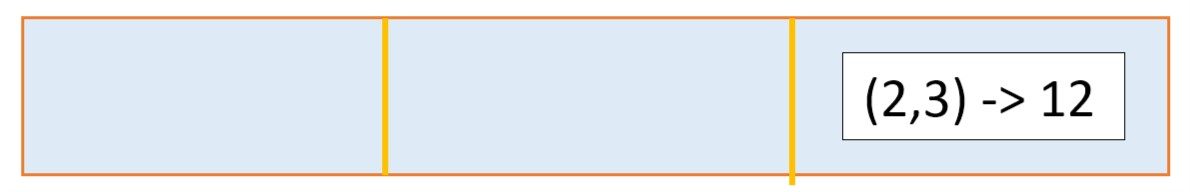Query 2:
(4,5)
F(4,5) = 24

LRU becomes: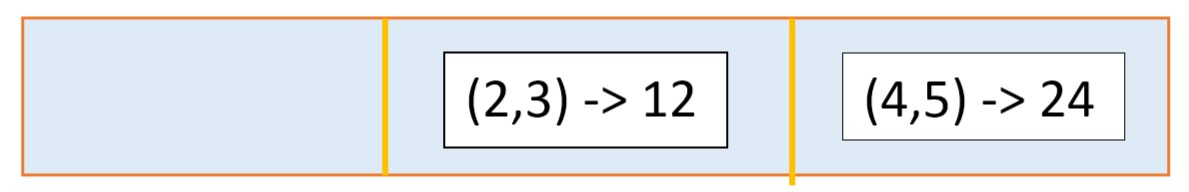Query 3:
(2,3)
Already calculated, Stored in Cache. So, you don't need to compute it again.

LRU becomes: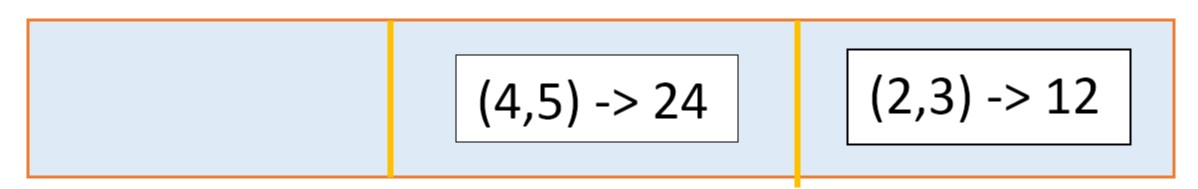Query 4:
(7,8)
F(7,8) = 42

LRU becomes: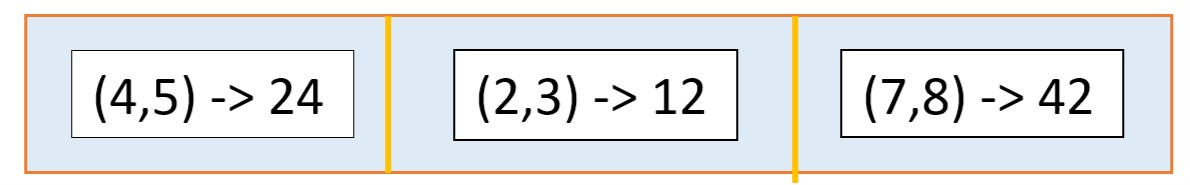(4,5) -> 24 is the last object present in the cache, if any new object comes it will be popped out from the cache.

Query 5:
(5,6)
F(5,6) = 30

LRU becomes: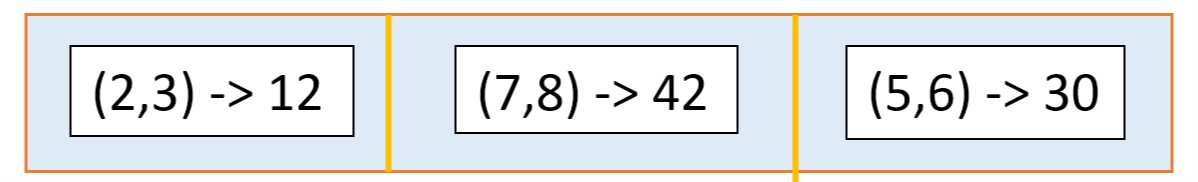Last Object (4,5) -> 24 popped out.

Query 6:
(4,5)
You will see that for (4,5) result was calculated earlier but isn't stored in Cache. So, you need to compute it again.
F(4,5) = 24

LRU becomes: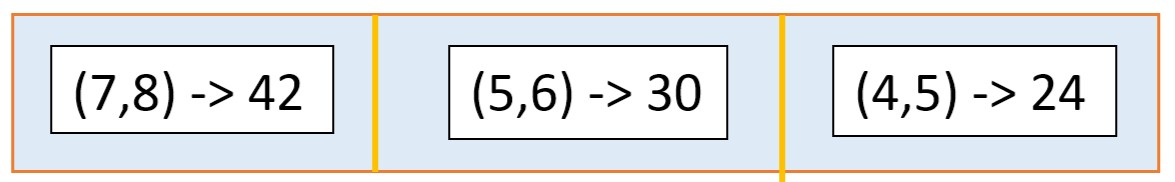Hoping that you had understood how LRU Cache works, Let's see how to use it in python.

## LRU Cache in Python

You can use LRU Cache using Python’s functools.lru_cache decorator.

Case 1: Calculating time for recursive calculation of equation for 1,000,000 times without using Cache.

``````import timeit

def solveEquation(x,y):
return 2*x + 4*y - 4

time = timeit.timeit('solveEquation(2,3)', globals=globals(), number=1000000)
``````

By running the above code you will get time as:

``````0.14199211914092302
``````

Case 2: Now calculating time for calculating equation using functools.lru_cache with a recursive function.

``````import timeit
import functools

@functools.lru_cache(maxsize=3)
def solveEquation(x,y):
return 2*x + 4*y - 4

time = timeit.timeit('solveEquation(2,3)', globals=globals(), number=1000000)
``````

By running the above code you will get time as:

``````0.08165495004504919
``````

By observing the Case-1 and Case-2, you will observe that using functools.lru_cache improves the time-performance of the computation.

Hoping that you had understood the difference between using functools.lru_cache and not using it.

Now, Let's breakdown the @functools.lru_cache(maxsize=128, typed=False) for a better understanding of how to use it.

### functools

functools is a python module used for higher-order functions: functions that act on or return other functions.

### lru_cache

lru_cache is a decorator applied directly to a user function to add the functionality of LRU Cache.

### maxsize

maxsize is the maximum number of objects you can store in a cache.
If you didn't pass maxsize as a parameter, then by default maxsize will be 128.
If maxsize is set to None, the LRU feature is disabled and the cache can grow without bound.

### typed

If typed is set to true, function arguments of different types will be cached separately. For example, f(3) and f(3.0) will be treated as distinct calls with distinct results.

### Functions

This decorator provides a cache_clear() function for clearing the cache.
Function cache_info() returns a named tuple showing hits, misses, maxsize, and currsize.

Hoping that you have understood the Cache and how to use it.